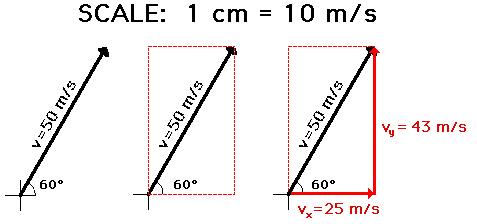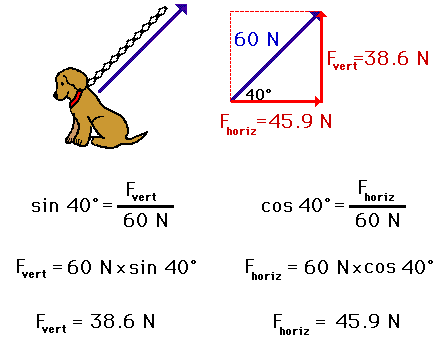Vectors - Motion and Forces in Two Dimensions - Lesson 1 - Vectors: Fundamentals and Operations

# Vector Resolution

As mentioned earlier in this lesson, any vector directed at an angle to the horizontal (or the vertical) can be thought of as having two parts (or components). That is, any vector directed in two dimensions can be thought of as having two components. For example, if a chain pulls upward at an angle on the collar of a dog, then there is a tension force directed in two dimensions. This tension force has two components: an upward component and a rightward component. As another example, consider an airplane that is displaced northwest from O'Hare International Airport (in Chicago) to a destination in Canada. The displacement vector of the plane is in two dimensions (northwest). Thus, this displacement vector has two components: a northward component and a westward component.

In this unit, we learn two basic methods for determining the magnitudes of the components of a vector directed in two dimensions. The process of determining the magnitude of a vector is known as vector resolution. The two methods of vector resolution that we will examine are

### Parallelogram Method of Vector Resolution

The parallelogram method of vector resolution involves using an accurately drawn, scaled vector diagram to determine the components of the vector. Briefly put, the method involves drawing the vector to scale in the indicated direction, sketching a parallelogram around the vector such that the vector is the diagonal of the parallelogram, and determining the magnitude of the components (the sides of the parallelogram) using the scale. If one desires to determine the components as directed along the traditional x- and y-coordinate axes, then the parallelogram is a rectangle with sides that stretch vertically and horizontally. A step-by-step procedure for using the parallelogram method of vector resolution is:

1. Select a scale and accurately draw the vector to scale in the indicated direction.
2. Sketch a parallelogram around the vector: beginning at the tail of the vector, sketch vertical and horizontal lines; then sketch horizontal and vertical lines at the head of the vector; the sketched lines will meet to form a rectangle (a special case of a parallelogram).
3. Draw the components of the vector. The components are the sides of the parallelogram. The tail of the components start at the tail of the vector and stretches along the axes to the nearest corner of the parallelogram. Be sure to place arrowheads on these components to indicate their direction (up, down, left, right).
4. Meaningfully label the components of the vectors with symbols to indicate which component represents which side. A northward force component might be labeled Fnorth. A rightward velocity component might be labeled vx; etc.
5. Measure the length of the sides of the parallelogram and use the scale to determine the magnitude of the components in real units. Label the magnitude on the diagram.

The step-by-step procedure above is illustrated in the diagram below to show how a velocity vector with a magnitude of 50 m/s and a direction of 60 degrees above the horizontal may be resolved into two components. The diagram shows that the vector is first drawn to scale in the indicated direction; a parallelogram is sketched about the vector; the components are labeled on the diagram; and the result of measuring the length of the vector components and converting to m/s using the scale. (NOTE: because different computer monitors have different resolutions, the actual length of the vector on your monitor may not be 5 cm.)### Trigonometric Method of Vector Resolution

The trigonometric method of vector resolution involves using trigonometric functions to determine the components of the vector. Earlier in lesson 1, the use of trigonometric functions to determine the direction of a vector was described. Now in this part of lesson 1, trigonometric functions will be used to determine the components of a single vector. Recall from the earlier discussion that trigonometric functions relate the ratio of the lengths of the sides of a right triangle to the measure of an acute angle within the right triangle. As such, trigonometric functions can be used to determine the length of the sides of a right triangle if an angle measure and the length of one side are known.

The method of employing trigonometric functions to determine the components of a vector are as follows:

1. Construct a rough sketch (no scale needed) of the vector in the indicated direction. Label its magnitude and the angle that it makes with the horizontal.
2. Draw a rectangle about the vector such that the vector is the diagonal of the rectangle. Beginning at the tail of the vector, sketch vertical and horizontal lines. Then sketch horizontal and vertical lines at the head of the vector. The sketched lines will meet to form a rectangle.
3. Draw the components of the vector. The components are the sides of the rectangle. The tail of each component begins at the tail of the vector and stretches along the axes to the nearest corner of the rectangle. Be sure to place arrowheads on these components to indicate their direction (up, down, left, right).
4. Meaningfully label the components of the vectors with symbols to indicate which component represents which side. A northward force component might be labeled Fnorth. A rightward force velocity component might be labeled vx; etc.
5. To determine the length of the side opposite the indicated angle, use the sine function. Substitute the magnitude of the vector for the length of the hypotenuse. Use some algebra to solve the equation for the length of the side opposite the indicated angle.
6. Repeat the above step using the cosine function to determine the length of the side adjacent to the indicated angle.

The above method is illustrated below for determining the components of the force acting upon Fido. As the 60-Newton tension force acts upward and rightward on Fido at an angle of 40 degrees, the components of this force can be determined using trigonometric functions.In conclusion, a vector directed in two dimensions has two components - that is, an influence in two separate directions. The amount of influence in a given direction can be determined using methods of vector resolution. Two methods of vector resolution have been described here - a graphical method (parallelogram method) and a trigonometric method.

### More Practice

Use the Components of a Vector widget below to resolve a vector into its components. Simply enter the magnitude and direction of a vector. Then click the Submit button to view the horizontal and vertical components. Use the widget as a practice tool.

Next Section:
Jump To Next Lesson:

Follow Us